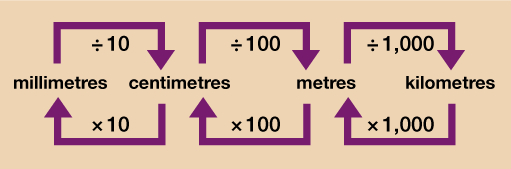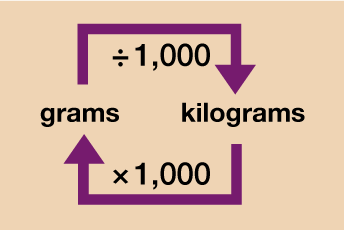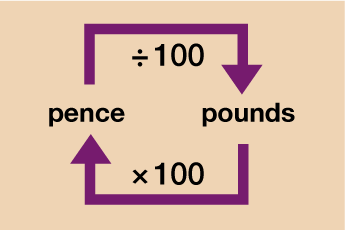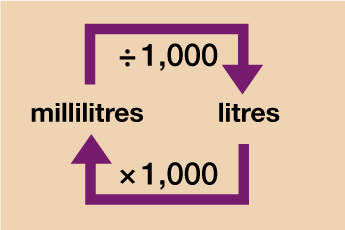Science, Maths & Technology

### Become an OU studentEveryday maths 2

Start this free course now. Just create an account and sign in. Enrol and complete the course for a free statement of participation or digital badge if available.

# 1.1 Converting units of measure in the same system

Imagine you are catering for a party and go to the wholesale shop to buy flour. You need 200 g of flour for each batch of cookies you will be making and need to make 30 batches. You can buy a 5 kg bag of flour but aren’t sure if that will be enough.

In order to work out if you have enough flour, you need both measurements to be in the same unit – kg or g – before you can do the calculation. For units of measure that are in the same system (so you are not dealing with converting cm and inches for example) it’s a simple process to convert one measurement into another. In most cases you will just need to multiply or divide by 10, 100 or 1,000. Take a look at the diagrams below which explain how to convert each type of measurement unit.

## LengthFigure _unit3.1.1 Figure 1 A conversion chart for length

## WeightFigure _unit3.1.2 Figure 2 A conversion chart for weight

## MoneyFigure _unit3.1.3 Figure 3 A conversion chart for money

## CapacityFigure _unit3.1.4 Figure 4 A conversion chart for capacity

You’ll learn how to convert between different currencies and different units of measure (kilograms (kg) to pounds (lb) for example) later in this section. For now, have a go at the activity below and test your conversion skills along with your problem solving ones!

## Activity _unit3.1.2 Activity 2: Solving conversion problems

1. A sunflower is 1.8 m tall. Over the next month, it grows a further 34 cm. How tall is the sunflower at the end of the month?

2. Peter is a long distance runner. In a training session he runs around the 400 m track 23 times. He wanted to run a distance of 10 km. How many more times does he need to run around the track to achieve this?

3. A water cooler comes with water containers that hold 12 l of water. The cups provided for use hold 150 ml. A company estimates that each of its 20 employees will drink 2 cups of water a day. How many 12 l bottles will be needed for each working week (Monday to Friday)?

4. David is a farmer and has 52 goats. He is given a bottle of wormer that contains 0.3 litres. The bottle comes with the instructions:

• ‘Use 4 ml of wormer for each 15 kg of body weight.’

The average weight of David’s goats is 21 kg and he wants to treat all of them. Does David have enough wormer?

1. 1 m = 100 cm so 1.8 × 100 = 180 cm

180 cm + 34 cm = 214 cm or 2.14 m

2. 400 m × 23 = 9200 m that Peter has run.

1 km = 1,000 m so, 10 km = 10 × 1,000 = 10,000 m

The difference between what Peter wants to run and what he has already run is:

• 10,000 – 9,200 = 800 m

• 800 ÷ 400 = 2

Peter needs to run another 2 laps of the track.

3. 1 litre = 1,000 ml so 12 l = 12 × 1,000 = 12,000 ml

Each employee will drink 2 × 150 ml = 300 ml a day

There are 20 employees so 300 × 20 = 6,000 ml for all employees per day

A working week is 5 days:

• 6,000 × 5 = 30,000 ml for all employees for the week

• 30,000 ÷ 12,000 = 2.5

They will need 2 and a half containers per week.

4. 1 litre = 1,000 ml so 0.3 litres = 0.3 × 1,000 = 300 ml of wormer in the bottle

52 goats at 21 kg each is 52 × 21 = 1092 kg total weight of goats

To find the number of 4 ml doses required do:

• 1092 ÷ 15 = 72.8

• 72.8 × 4 ml = 291.2 ml of wormer needed.

Yes, David has enough wormer.

Hopefully you will now be feeling confident with converting units of measure within the same system. You’ll need to know how to do this and be able to remember how to convert from one to another by multiplying or dividing.

If you are being asked to convert between litres and gallons or US dollars and British pounds however, you won’t be expected to know the conversion rate, you’ll be given it in the question. The next section will show you how to use these conversion rates and you’ll be able to practise solving problems that require you to do this.

## Summary

In this section you have learned:

• that different units are used for measurement

• unit selection depends on the item or object being measured

• how to convert between units in the same system.